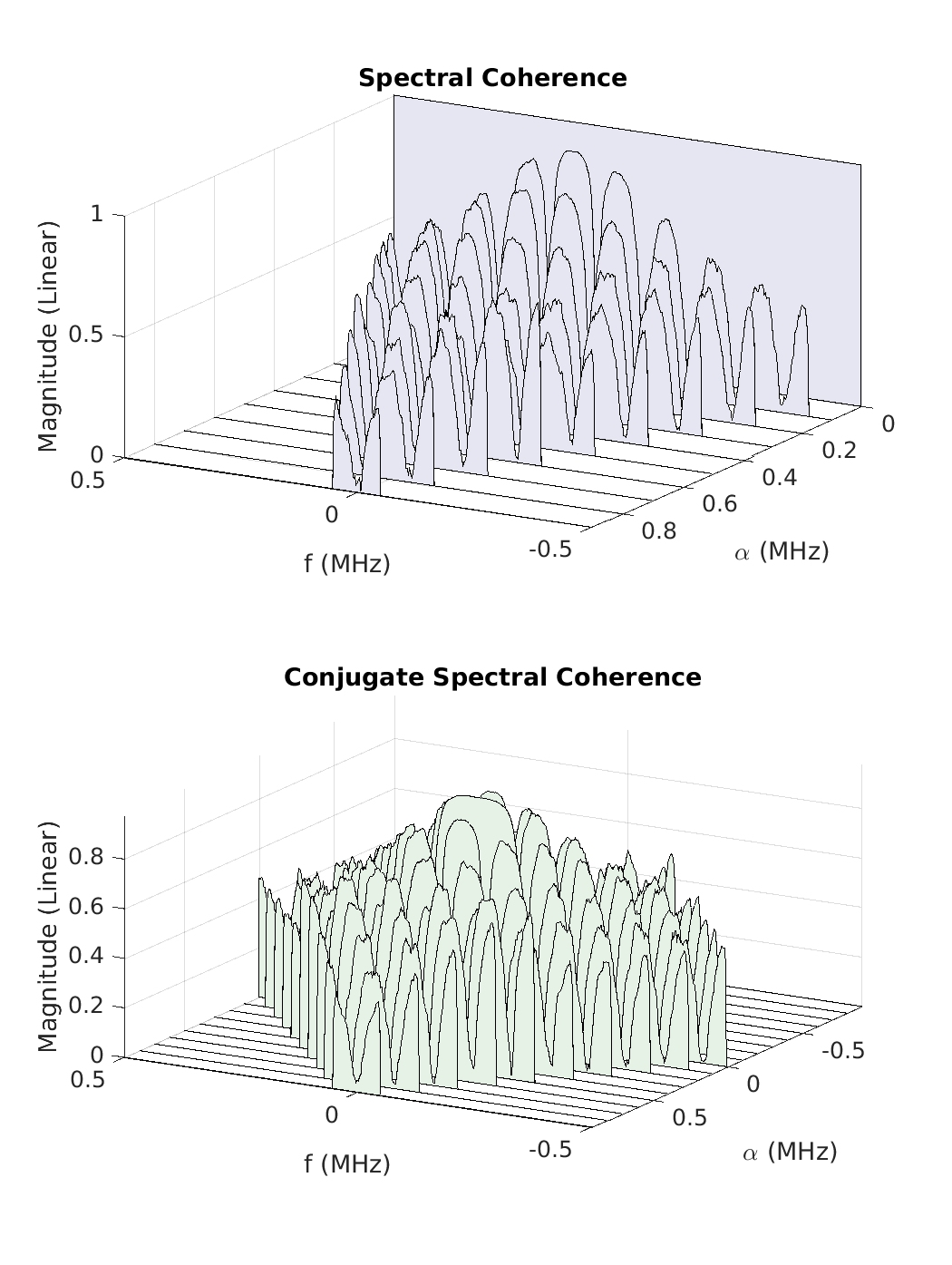# The Spectral Coherence Function

In this post I introduce the spectral coherence function, or just coherence. It deserves its own post because the coherence is a useful detection statistic for blindly determining significant cycle frequencies of arbitrary data records.

Let’s start with reviewing the standard correlation coefficient$\rho$ defined for two random variables$X$ and$Y$ as$\rho = \displaystyle \frac{E[(X - m_X)(Y - m_Y)]}{\sigma_X \sigma_Y}, \hfill (1)$

where$m_X$ and$m_Y$ are the mean values of$X$ and$Y$, and$\sigma_X$ and$\sigma_Y$ are the standard deviations of$X$ and$Y$. That is,$m_X = E[X] \hfill (2)$$m_Y = E[Y] \hfill (3)$$\sigma_X^2 = E[(X-m_X)^2] \hfill (4)$$\sigma_Y^2 = E[(Y-m_Y)^2] \hfill (5)$

So the correlation coefficient is the covariance between$X$ and$Y$ divided by the geometric mean of the variances of$X$ and$Y$.

Now consider the spectral correlation function,$S_x^\alpha(f) = \displaystyle \lim_{T\rightarrow\infty} \frac{1}{T} E[ X_T(t, f+\alpha/2) X_T^* (t, f-\alpha/2)], \hfill (6)$

where the expectation operator$E[\cdot]$ can be either a time average or the ensemble average used in a stochastic-process formulation. For each finite$T$, we can consider the quantity$\displaystyle \frac{1}{T} E[X_T(t, f+\alpha/2) X_T^* (t, f-\alpha/2)], \hfill (7)$

which is the correlation between the two random variables$\displaystyle \frac{1}{T^{1/2}} X_T(t, f+\alpha/2)$ and$\displaystyle \frac{1}{T^{1/2}} X_T(t, f-\alpha/2)$. To form the correlation coefficient, we need the variances for these two random variables,$\sigma_1^2(T) = E[\displaystyle\frac{1}{T} \left| X_T(t, f+\alpha/2) \right|^2] \hfill (8)$

and$\sigma_2^2(T) = E[\displaystyle\frac{1}{T} \left| X_T(t, f-\alpha/2) \right|^2]. \hfill (9)$

Here we are assuming that the two variables have zero means, which is true when there is no additive sine-wave component in the data with frequency$f\pm \alpha/2$.

For finite$T$, then, we can form the correlation coefficient$\rho(T) = \displaystyle \frac{\frac{1}{T} E[X_T(t, f+\alpha/2) X_T^* (t, f-\alpha/2)]}{[\sigma_1^2(T) \sigma_2^2(T)]^{1/2}}. \hfill (10)$

Now, as$T\rightarrow \infty$, the numerator of$\rho(T)$ converges to the spectral correlation function$S_x^\alpha(f)$ and$\displaystyle \lim_{T\rightarrow\infty} \sigma_1^2(T) = S_x^0(f+\alpha/2) \hfill (11)$

and$\displaystyle \lim_{T\rightarrow\infty} \sigma_2^2(T) = S_x^0(f-\alpha/2). \hfill (12)$

If the limit of the quotient exists, then the correlation coefficient is given by$\rho = C_x^\alpha(f) = \displaystyle\frac{S_x^\alpha(f)}{[S_x^0(f+\alpha/2)S_x^0(f-\alpha/2)]^{1/2}}. \hfill (13)$

We call this function the spectral coherence. A similar argument holds for the conjugate spectral coherence,$C_{x^*}^\alpha(f) = \displaystyle\frac{S_{x^*}^\alpha(f)}{[S_x^0(f+\alpha/2)S_x^0(\alpha/2-f)]^{1/2}}. \hfill (14)$

Since the coherence is a valid correlation coefficient, its magnitude will be less than or equal to one, and since the involved random variables are complex-valued, in general, so is the spectral correlation function and the coherence. Therefore, the coherence lies in the closed unit disk in the complex plane.

Here is an FSM-based estimate of the coherence for our rectangular-pulse BPSK signal:The data-block length is$65536$ samples and the frequency resolution (width of$g(f)$ in the FSM) is set to$0.01$ (one percent of the sampling rate, which here is$1.0$) for both the numerator spectral correlation function and the denominator PSDs.

In practice, of course, the numerator and denominator of the coherence are estimates corresponding to finite-duration data blocks. The tricky part of estimating the coherence involves good selection of the estimator for the denominator PSDs$S_x^0(f\pm\alpha/2)$. If the PSDs are not well resolved, or contain zeros, the coherence quotient can be numerically unstable or erroneous.I'm a signal processing researcher specializing in cyclostationary signal processing (CSP) for communication signals. I hope to use this blog to help others with their cyclo-projects and to learn more about how CSP is being used and extended worldwide.

Posted on

## 2 thoughts on “The Spectral Coherence Function”

1.Chen says:

I cannot help asking you how to estiamte the denominator PSDs? I supposed that the FAM or SSCA based SCF estimates are obtained. Then I think FFT algorithm is a must to avoid computational complexity when estimating PSDs. I have tried a lot but I cannot present a detail solution to estimate PSDs furthermore.
1.Chad Spooner says: